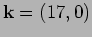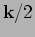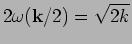Next: Amplitude and phase evolution. Up: Results Previous: Wave-amplitude probability density function

## Frequency properties.

We examine the frequency properties of waves by performing the time-Fourier transform at each fixed wavenumber. A typical plot, for, is shown in Figures 6. Our first observation is that we always see two peaks - the bigger one at the linear frequency and a smaller peak at a shifted frequency. We interpret the second peak as a nonlinear effect since there is no frequency shift in the linear system.

 [width=10cm]freq_k17.eps

Also, it appears that the measured ratio of squares of the peak frequencies is approximately equal to 2 for all wavenumbers (within 10% accuracy). This can be explained by the nonlinear term in the canonical transformation (9), e.g.-term which is quadratic with respect to the wave amplitude. In particular, the modemakes contribution to this term which oscillates at frequencywhich appears to coincide with the second peak's frequency. Thus we see that contribution ofdominates in the nonlinear term of the canonical transformation.

The two-frequency character at each wavenumber has an interesting relation to the amplitude and phase dynamics as will be seen in the next section.Next: Amplitude and phase evolution. Up: Results Previous: Wave-amplitude probability density function
Dr Yuri V Lvov 2007-01-16